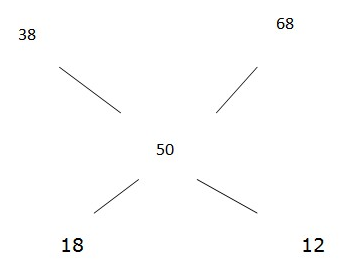# IBPS PO Prelims Quantitative Aptitude Questions 2021 (Day-05)

Dear Aspirants, Our IBPS Guide team is providing new series of Quantitative Aptitude Questions for IBPS PO Prelims 2021 so the aspirants can practice it on a daily basis. These questions are framed by our skilled experts after understanding your needs thoroughly. Aspirants can practice these new series questions daily to familiarize with the exact exam pattern and make your preparation effective.

Start Quiz

Mensuration

1) A cone and a sphere have the same radius of 15 cm. Find the height of the cone, if the volumes of the cone & that of sphere are equal.

A.60 cm

B.50 cm

C.40 cm

D.30 cm

E.20 cm

Partnership

2) Rakhi, Sabita and Rani started the business and the investment period in the ratio of 5:4:6 respectively. At the end of the business, the ratio of the profit shares of Rakhi, Sabita and Rani is 2:3:4 respectively, find the ratio of the investment of Rakhi, Sabita and Rani?

A.24:45:40

B.21:43:40

C.22:41:40

D.22:43:40

E.None of these

Permutation and combination

3) In how many different ways can the letters of the word “CORONA” be arranged?

A.720

B.360

C.180

D.280

E.1440

Trains

4) Two trains A and B is running on parallel tracks in the same direction at the speed of 45 kmph and 60 kmph respectively. If train B crosses train A in 144 seconds and the length of trains are equal, then find the length of each train?

A.240 m

B.200 m

C.280 m

D.300 m

E.360 m

SI AND CI

5) Rahul lends a part of Rs.4000 at 12% simple interest scheme and the remaining amount at 10% simple interest. If the total interest earned after 2 years is Rs.896, then find the sum lent at 12%?

A.Rs.1800

B.Rs.2200

C.Rs.2400

D.Rs.2500

E.None of these

probability

6) A box contains 3 red balls, 4 green balls and 8 black balls. If 3 balls are drawn at random, then what is the probability of that balls are black?

A.7/65

B.6/65

C.1/13

D.8/65

E.4/65

Boat and stream

7) A boat can cover 60 km in 5 hours in still water. How long will it take to cover the same distance in upstream, if the speed of water is 1/6th of the speed of boat?

A.5

B.20

C.15

D.12

E.none of these

Income and expenditure

8) The average monthly savings of Mahesh for first 4 months are 2000, for next 5 months are 1000 and for next 3 months are 3000. If his total expenditure for the whole year is 50,000, find his monthly income.

A.5000

B.6000

C.7500

D.4500

E.3500

Mixture and allegation

9) In what ratio two types of tea Rs.38/kg and Rs.68/kg should be mixed so that after selling the mixture at Rs.60/kg the shopkeeper gains 20%.

A.3:2

B.2:3

C.1:3

D.3:1

E.5:3

Ages

10) Vanu’s present age is four times of Soham’s age and 2/3rd of Rohan’s age. If the age difference of the Rohan and Soham is 50 years. Find the age of Vanu after 5 years.

A.25

B.55

C.35

D.45

E.none of these

1/3 π r2h = 4/3 π r3

= > h = 4r

= > h= 4*15= 60 cm

Investment ratio of Rakhi, sabita and Rani = 2/5:3/4:4/6

= 24:45:40

Number of letters = 6

Required ways = 6!/2! = 360

Length of train = x

2x = (60 – 45) * 5/18 * 144

x = 300 m

Amount lent at 12% = x

Amount lent at 10% = 4000 – x

x * 12 * 2/100 + (4000 – x) * 2 * 10/100 = 896

24x + 80000 – 20x = 89600

x = 2400

Required probability = 8C3/15C3

= (8 * 7 * 6)/(15 * 14 * 13)

= 8/65

The speed of boat in still water = 60/5 = 12kmph

The speed of water = 12/6 = 2kmph

Required answer= 60/(12-2) = 6 hours

Savings in first 4 months = 2000*4=8000

Savings in next 5 months= 1000*5 = 5000

Savings in last 3 months = 3000*3=9000

Total savings= (8000+5000+9000) = 22000

Total expenditure of a year= 50,000

Total income of a year= 50,000+22000 = 72,000

Monthly income=72000/12=6000

The cp of the mixture = 60*100/120 = 50 rs/kgRequired ratio = 18:12 = 3:2

The ratio of the age of Vanu, Soham and Rohan = 4:1:6

= > 5’s = 50

= > 4’s = 40

Vanu’s present age = 40

After 5 years, age will be = 40+5 =45

5 1 vote
Rating